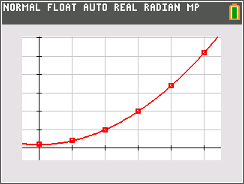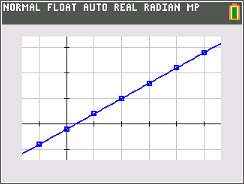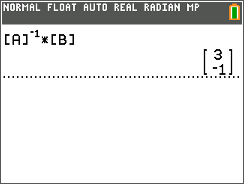••• ##### Device
• TI-84 Plus
• TI-84 Plus Silver Edition
•TI-84 Plus C Silver Edition
•TI-84 Plus CE
• ##### Software

TI Connect™ CE

# Algebra II: Count the Differences

by Texas Instruments#### Overview

Students are given data, asked to find the finite differences, and then use this to find a polynomial that models the data.

#### Key Steps

•Students begin by finding the first-order differences of a linear relation to make the connection between finding slope and finite differences.

•Then, students will find second- and third-order finite differences in order to find a polynomial that models the given data.

•As an extension, students will use matrix equations to find polynomials that model the data from this activity.